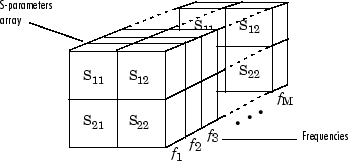## S-Parameter Notation

### Define S-Parameters

RF Toolbox™ software uses matrix notation to specify S-parameters. The indices of an S-parameter matrix correspond to the port numbers of the network that the data represent. For example, to define a matrix of 50-ohm, 2-port S-parameters, type:

```s11 = 0.61*exp(j*165/180*pi); s21 = 3.72*exp(j*59/180*pi); s12 = 0.05*exp(j*42/180*pi); s22 = 0.45*exp(j*(-48/180)*pi); s_params = [s11 s12; s21 s22];```
RF Toolbox functions that operate on `s_params` assume:

• `s_params(1,1)` corresponds to the reflection coefficient at port 1, S11.

• `s_params(2,1)` corresponds to the transmission coefficient from port 1 to port 2, S21.

• `s_params(1,2)` corresponds to the transmission coefficient from port 2 to port 1, S12.

• `s_params(2,2)` corresponds to the reflection coefficient at port 2, S22.

RF Toolbox software also supports three-dimensional arrays of S-parameters. The third dimension of an S-parameter array corresponds to S-parameter data at different frequencies. The following figure illustrates this convention.### Refer to S-Parameters Using Character Vector

RF Toolbox software uses character vector to refer to S-parameters in plotting and calculation methods, such as `plot`. These character vector have one of the following two forms:

• `'Snm'` — Use this syntax if `n` and `m` are both less than 10.

• `'Sn,m'` — Use this syntax if one or both are greater than 10. `'Sn,m'` is not a valid syntax when both `n` and `m` are less than 10.

The indices `n` and `m` are the port numbers for the S-parameters.

Most toolbox objects only analyze 2-port S-parameters. The following objects analyze S-parameters with more than two ports:

You can get 2-port parameters from S-parameters with an arbitrary number of ports using one or more of the following steps:

• Extract 2-port S-parameters from N-port S-parameters.

• Convert single-ended 4-port parameters to differential 2-port parameters.